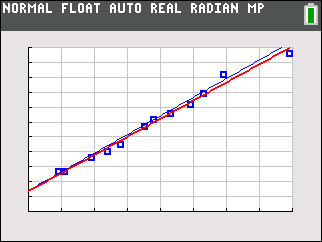•• ##### Device
• TI-84 Plus
•TI-84 Plus CE

TI Connect™

• ##### Report an Issue

SA and NT: You Are What You Eat
by Texas Instruments#### Overview

Students are introduced to modeling linear data through an investigation of comparing grams of fat and calories in fast food hamburgers.

#### Key Steps

•Students use linear regression to investigate the relationship between the number of calories and the number of grams of fat in fast food hamburgers.

•Students set up and equation to relate calories vs. grams of fat by plotting the data as a scatter plot, then find the line of best fit.

•Students compare the equation found with the regression equation. They examine how similar or different the equations are.